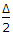# Civil Engineering - Surveying

Exercise : Surveying - Section 8
1.
Pick up the correct statement from the following :
The directions of plumb lines suspended at different points in a survey are not strictly parallel
In surveys of small extent, the effect of curvature may be ignored and the level surface of the earth is assumed as horizontal
In surveys of large extent, the effect of curvature of the earth must be considered
All the above.
Explanation:
No answer description is available. Let's discuss.

2.
If Δ is the angle of deflection of a simple curve of radius R, the length of its long chord, is
R cos2R cosR sin2R sin.
Explanation:
No answer description is available. Let's discuss.

3.
In geodetic surveys higher accuracy is achieved, if
curvature of the earth surface is ignored
curvature of the earth surface is taken into account
angles between the curved lines are treated as plane angles
none of these.
Explanation:
No answer description is available. Let's discuss.

4.
Determining the difference in elevation between two points on the surface of the earth, is known as
levelling
simple levelling
differential levelling
longitudinal levelling.